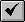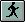English

How To: Convert view graphics into a shapefile using Avenue

Summary

Instructions provided describe how to use Avenue to convert graphics, found in a view, to features in a shapefile.

Procedure

Run the following script on a view which contains graphics. If there are selected graphics, this script converts only those graphics. If there are no selected graphics, it converts all graphics of the selected type.

1. Open a new script window.

A. Activate the Project window.
B. Click the Scripts icon.
C. Click New.

2. Copy the code into the new script window.

Code:
'-- Scriptgra2shp.ave
'-- Converts all view graphics of the selected feature type into a shapefile,
'-- then adds that shapefile to the View.

theView=av.getactivedoc
theGPL=theView.getgraphics.findallbyclass(GraphicShape)
if (theGPL = nil) then msgbox.error("No graphic shapes in view","") return nil
end

theFTL={"Point", "Polyline", "Polygon"}
aShapeType=msgbox.listasstring(theFTL, "Select the type of graphics you want"+
"converted into a shapefile", "Choose a feature type")
if (aShapeType = nil) then return nil end

if (aShapetype = "point") then
theclass = point
elseif (aShapeType = "polyline") then
theclass = polyline
elseif (aShapeType = "polygon") then
theclass = polygon
end

theCLoF={}
for each g in theGPL
if ((g.getshape.is(rect)) or (g.getshape.is(circle))) then
g = graphicshape.make(g.getshape.aspolygon)
end
if (g.getshape.Is(theclass)) then
end
end

if (theCLoF.count = 0) then msgbox.error("No "+aShapeType+" graphic features in"+
"view","") return nil end

tmpfn = "c:\".asfilename.maketmp("test","shp")
theOutFileName=FileDialog.Put(tmpfn,"*.shp","Create new shapefile from simple"+
"graphics")
theFtab=Ftab.MakeNew(theOutFileName, theclass)
theIDfld=Field.Make("myID", #FIELD_LONG, 10, 0)
theShapeFld=theFtab.FindField("Shape")
theFldList={theIDfld}

for each fea in theCLoF